# Linear Equations

Parent topic:
Equations
•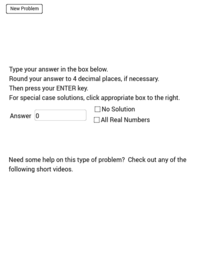### solving linear equations

Book

Verna Ashcraft

•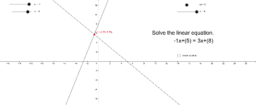### Solving Linear Equations

Book

Monica Woo

•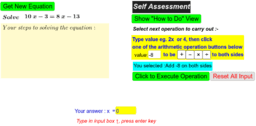### Solving Linear Equations

Book

seacat

•### Algebra Linear equations

Book

Kgibson

•### algebra linear equations

Book

•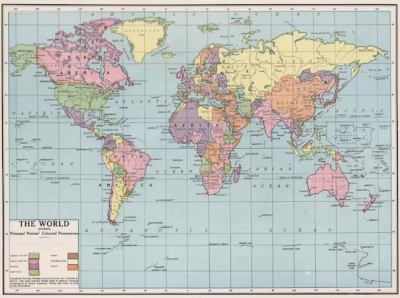### Coordinate Geometry

Book

Simona Riva

•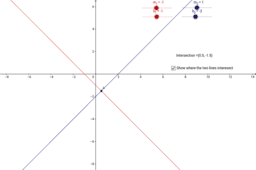### Systems of Linear Equations

Book

hnees

•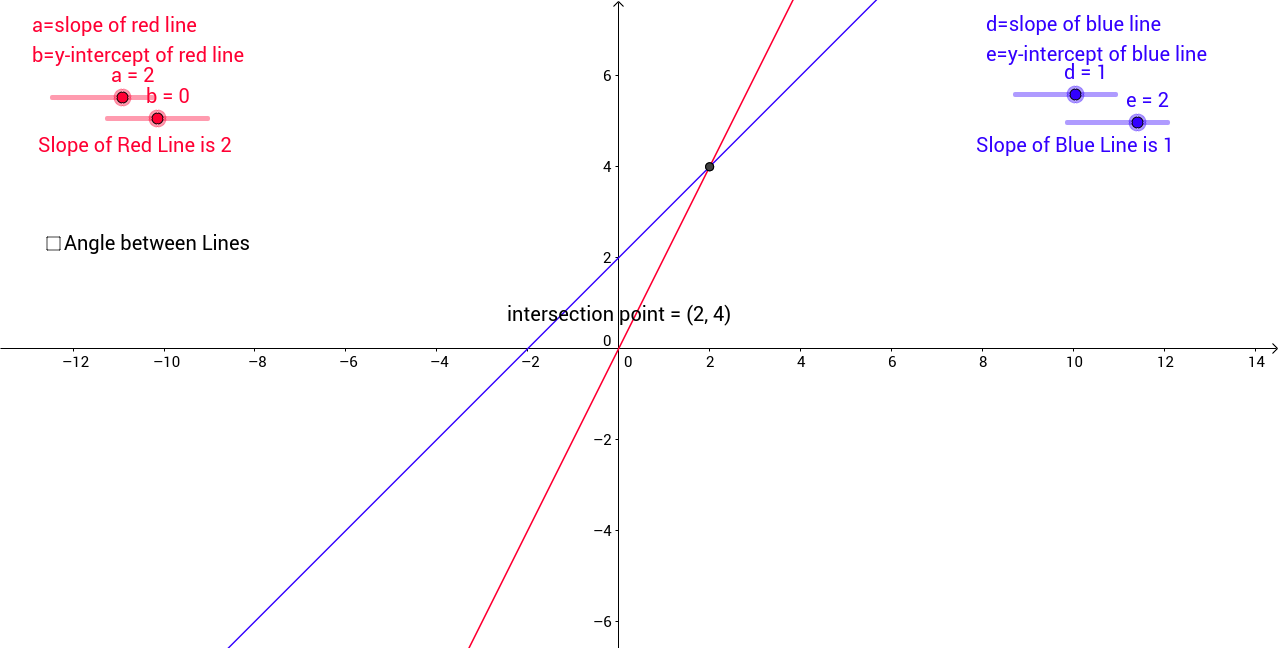### System of Linear Equations

Activity

wsligh

•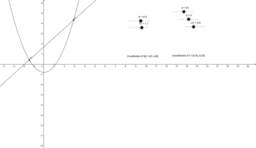### Solving Simultaneous Non-Linear Equations

Activity

Selina Chan

•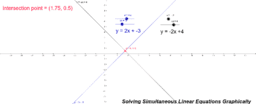### Solving Simultaneous Linear Equations Graphically

Activity

Selina Chan

•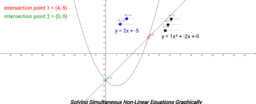### Solving Simultaneous Non-Linear Equations Graphically

Activity

Selina Chan

•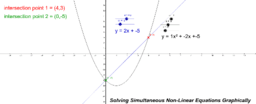### Solving Simultaneous Non-Linear Equations Graphically

Activity

Selina Chan

•### Solving Linear Equations

Activity

seacat

•### Solve Simple Linear Equations ( Balance Concept)

Activity

Lew W.S.

•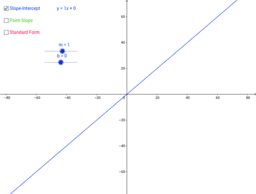### Exploring Linear Equations

Activity

GeoGebra Materials Team

•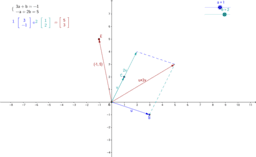### Systems of Linear Equations

Activity

Ana D Todorova

•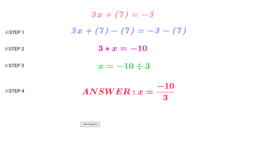### Linear Equations of the form ax+b=c. Level 1.

Activity

hasulkin2

•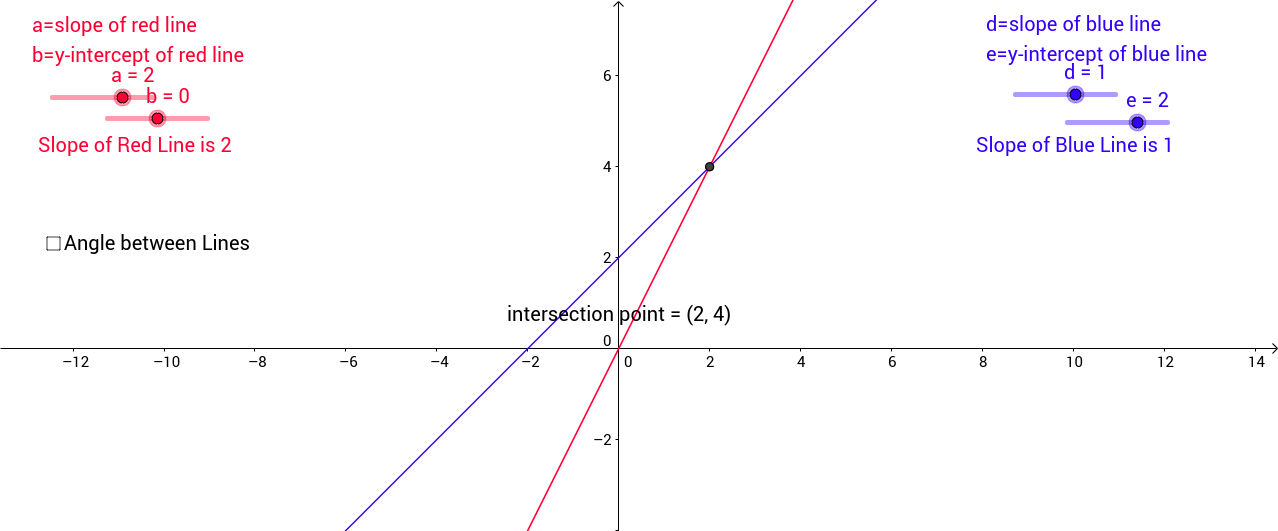### ACCESS - System of Linear Equations

Activity

Accesscd

•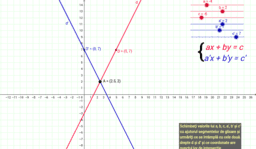### System of equations

Activity

•### Exploring Linear Equations

Activity

mcsmith77

•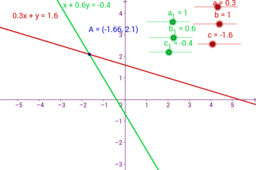### solve a pair of linear equations in two variables.

Activity

Mathguru

•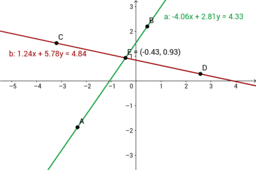### solve a pair of linear equations in two variables.

Activity

Mathguru

•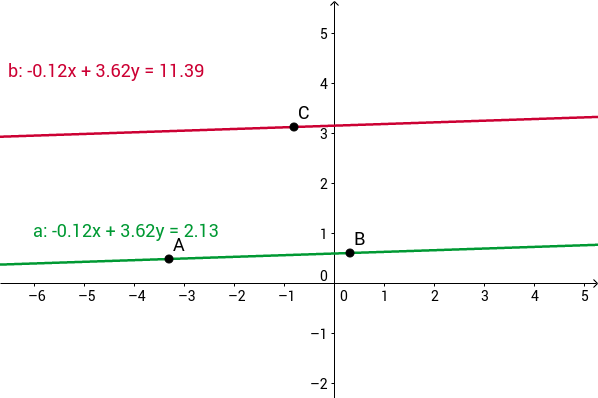### solve a pair of linear equations in two variables.

Activity

Mathguru

•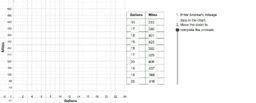### CCGPS CA 4.2.2 Example 1

Activity

Walch Education